# FirstOrder Logic Pros and cons of propositional logic

• Slides: 26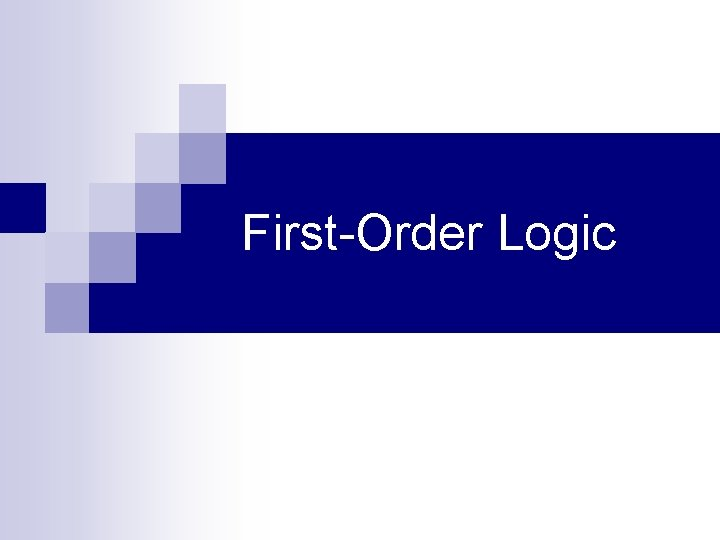First-Order Logic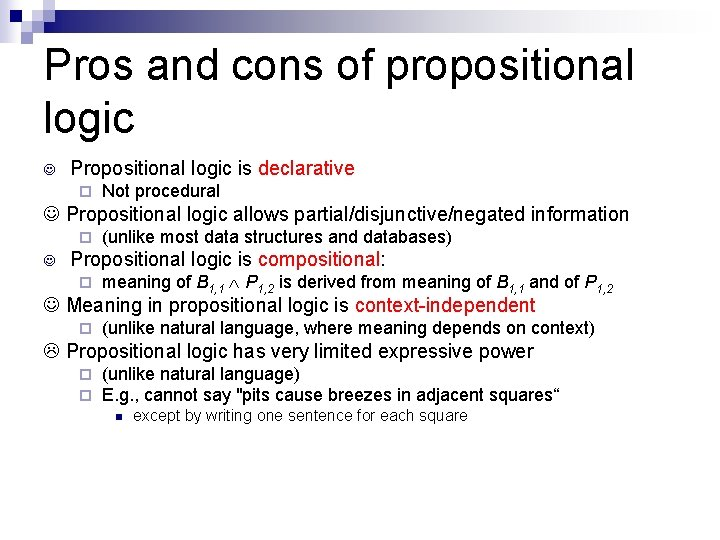Pros and cons of propositional logic Propositional logic is declarative ¨ Not procedural Propositional logic allows partial/disjunctive/negated information ¨ (unlike most data structures and databases) Propositional logic is compositional: ¨ meaning of B 1, 1 P 1, 2 is derived from meaning of B 1, 1 and of P 1, 2 Meaning in propositional logic is context-independent ¨ (unlike natural language, where meaning depends on context) Propositional logic has very limited expressive power ¨ ¨ (unlike natural language) E. g. , cannot say "pits cause breezes in adjacent squares“ n except by writing one sentence for each squareFirst-order logic n n Whereas propositional logic assumes the world contains facts, First-order logic (like natural language) assumes the world contains ¨ Objects: people, houses, numbers, colors, baseball games, wars, … ¨ Relations: red, round, prime, brother of, bigger than, part of, comes between, … ¨ Functions: father of, best friend, one more than, plus, …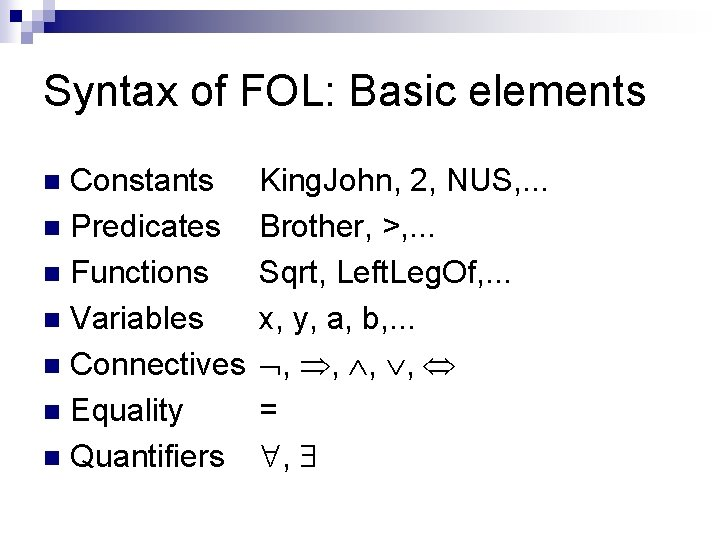Syntax of FOL: Basic elements Constants n Predicates n Functions n Variables n Connectives n Equality n Quantifiers n King. John, 2, NUS, . . . Brother, >, . . . Sqrt, Left. Leg. Of, . . . x, y, a, b, . . . , , = ,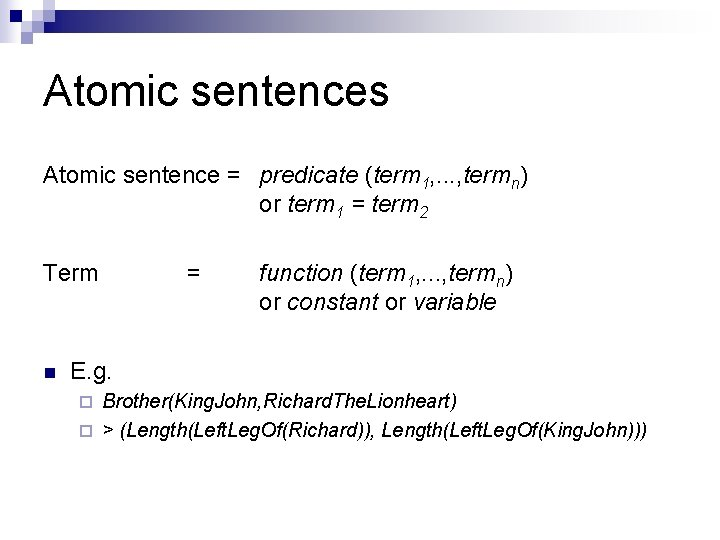Atomic sentences Atomic sentence = predicate (term 1, . . . , termn) or term 1 = term 2 Term n = function (term 1, . . . , termn) or constant or variable E. g. Brother(King. John, Richard. The. Lionheart) ¨ > (Length(Left. Leg. Of(Richard)), Length(Left. Leg. Of(King. John))) ¨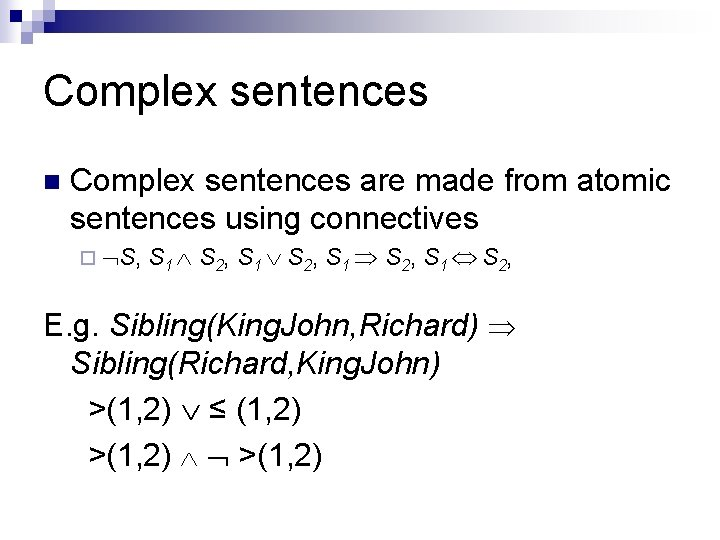Complex sentences n Complex sentences are made from atomic sentences using connectives ¨ S, S 1 S 2, E. g. Sibling(King. John, Richard) Sibling(Richard, King. John) >(1, 2) ≤ (1, 2) >(1, 2)Truth in first-order logic n n n Sentences are true with respect to a model and an interpretation Model contains objects (domain elements) and relations among them Interpretation specifies referents for constant symbols → predicate symbols → function symbols → n objects relations functional relation An atomic sentence predicate(term 1, . . . , termn) is true iff the objects referred to by term 1, . . . , termn are in the relation referred to by predicate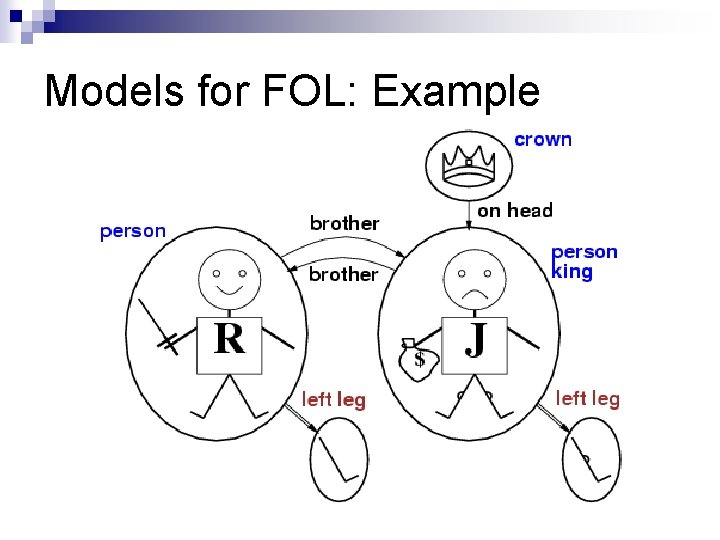Models for FOL: ExampleUniversal quantification n <variables> <sentence> Everyone at SCU is smart: x At(x, SCU) Smart(x) x P is true in a model m iff P is true with x being each possible object in the model Roughly speaking, equivalent to the conjunction of instantiations of P . . . At(King. John, NUS) Smart(King. John) At(Richard, NUS) Smart(Richard) At(NUS, NUS) Smart(NUS)A common mistake to avoid n Typically, is the main connective with n Common mistake: using as the main connective with : x At(x, SCU) Smart(x) means “Everyone is at SCU and everyone is smart”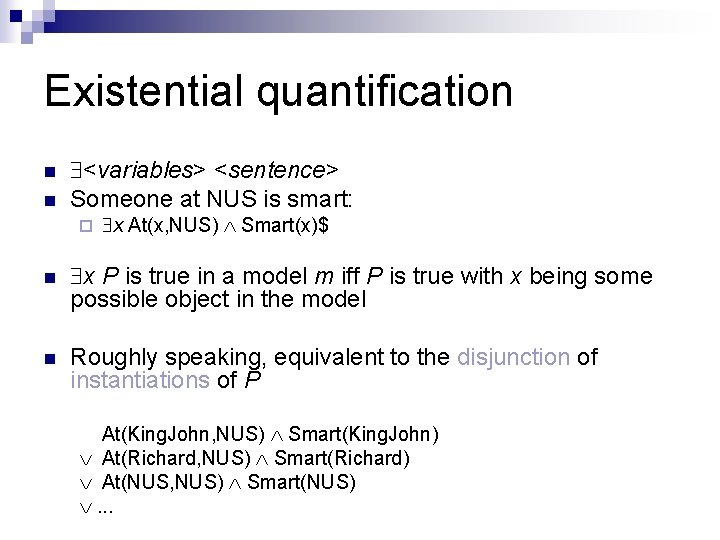Existential quantification n n <variables> <sentence> Someone at NUS is smart: ¨ x At(x, NUS) Smart(x)\$ n x P is true in a model m iff P is true with x being some possible object in the model n Roughly speaking, equivalent to the disjunction of instantiations of P At(King. John, NUS) Smart(King. John) At(Richard, NUS) Smart(Richard) At(NUS, NUS) Smart(NUS) . . .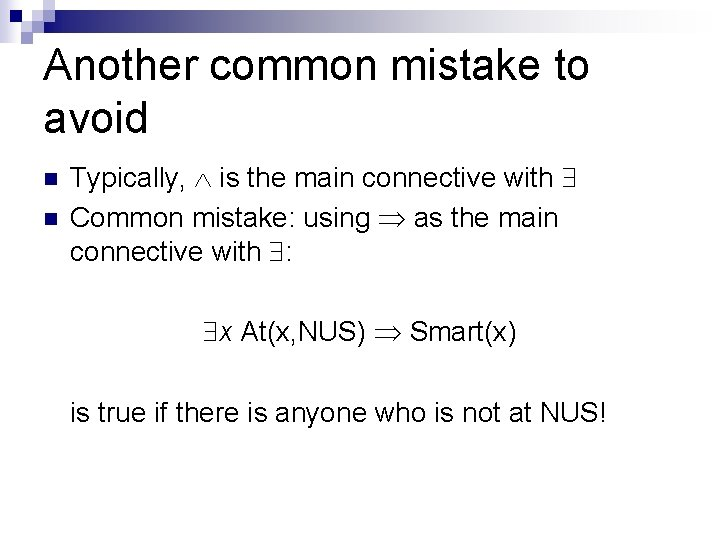Another common mistake to avoid n n Typically, is the main connective with Common mistake: using as the main connective with : x At(x, NUS) Smart(x) is true if there is anyone who is not at NUS!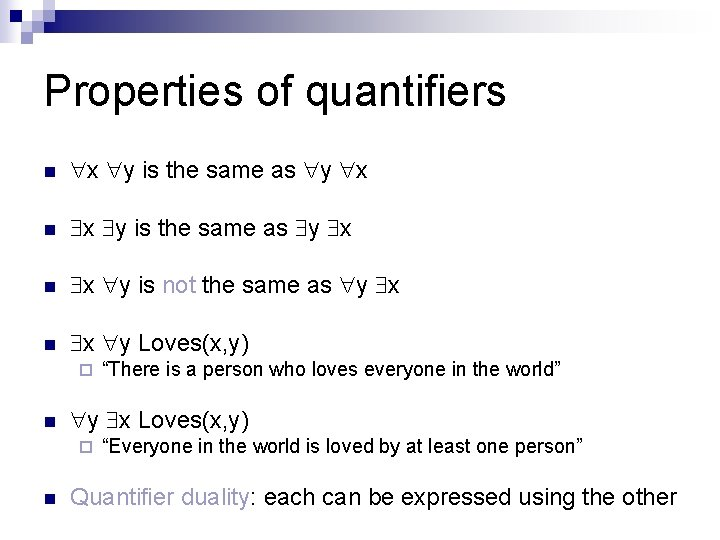Properties of quantifiers n x y is the same as y x n x y is not the same as y x n x y Loves(x, y) ¨ n y x Loves(x, y) ¨ n “There is a person who loves everyone in the world” “Everyone in the world is loved by at least one person” Quantifier duality: each can be expressed using the other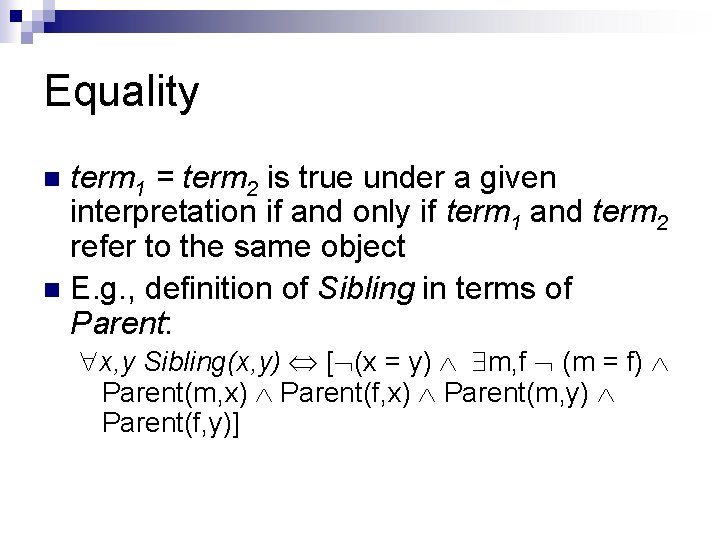Equality term 1 = term 2 is true under a given interpretation if and only if term 1 and term 2 refer to the same object n E. g. , definition of Sibling in terms of Parent: n x, y Sibling(x, y) [ (x = y) m, f (m = f) Parent(m, x) Parent(f, x) Parent(m, y) Parent(f, y)]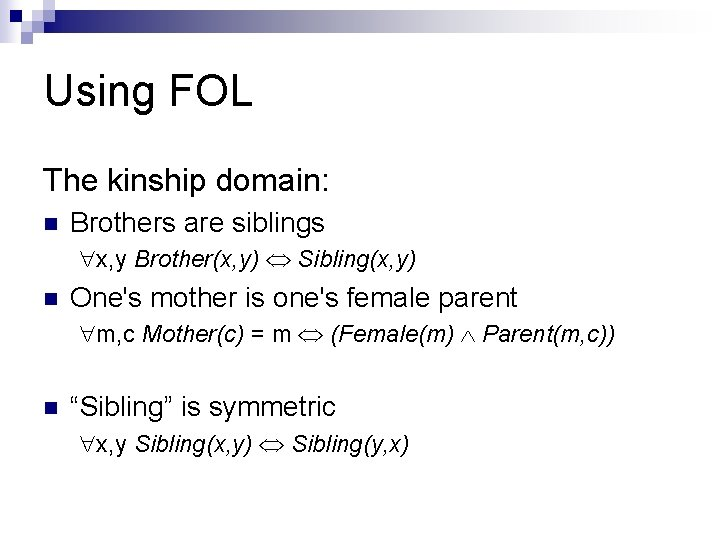Using FOL The kinship domain: n Brothers are siblings x, y Brother(x, y) Sibling(x, y) n One's mother is one's female parent m, c Mother(c) = m (Female(m) Parent(m, c)) n “Sibling” is symmetric x, y Sibling(x, y) Sibling(y, x)Using FOL The set domain: n s Set(s) (s = {} ) ( x, s 2 Set(s 2) s = {x|s 2}) n x, s {x|s} = {} n x, s x s s = {x|s} n x, s x s [ y, s 2} (s = {y|s 2} (x = y x s 2))] n s 1, s 2 s 1 s 2 ( x x s 1 x s 2) n s 1, s 2 (s 1 = s 2) (s 1 s 2 s 1) n x, s 1, s 2 x (s 1 s 2) (x s 1 x s 2)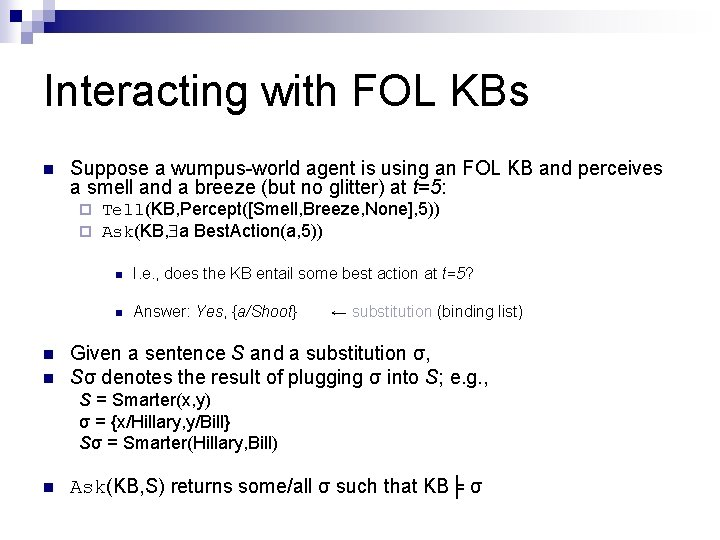Interacting with FOL KBs n Suppose a wumpus-world agent is using an FOL KB and perceives a smell and a breeze (but no glitter) at t=5: ¨ ¨ n n Tell(KB, Percept([Smell, Breeze, None], 5)) Ask(KB, a Best. Action(a, 5)) n I. e. , does the KB entail some best action at t=5? n Answer: Yes, {a/Shoot} ← substitution (binding list) Given a sentence S and a substitution σ, Sσ denotes the result of plugging σ into S; e. g. , S = Smarter(x, y) σ = {x/Hillary, y/Bill} Sσ = Smarter(Hillary, Bill) n Ask(KB, S) returns some/all σ such that KB╞ σ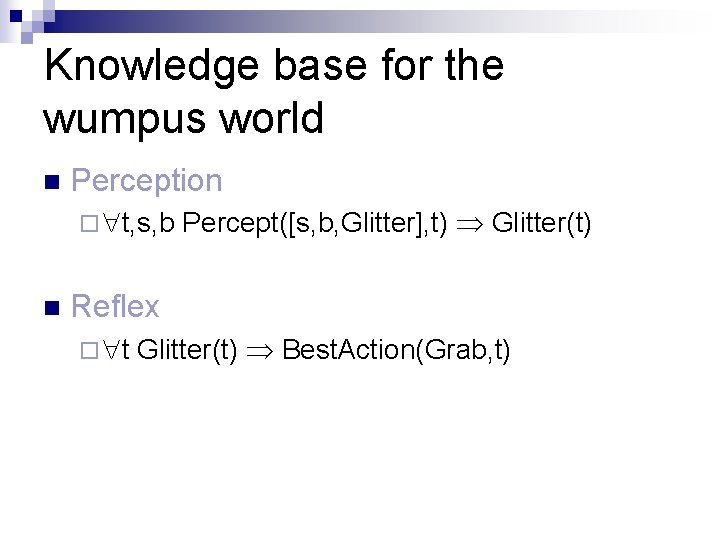Knowledge base for the wumpus world n Perception ¨ t, s, b n Percept([s, b, Glitter], t) Glitter(t) Reflex ¨ t Glitter(t) Best. Action(Grab, t)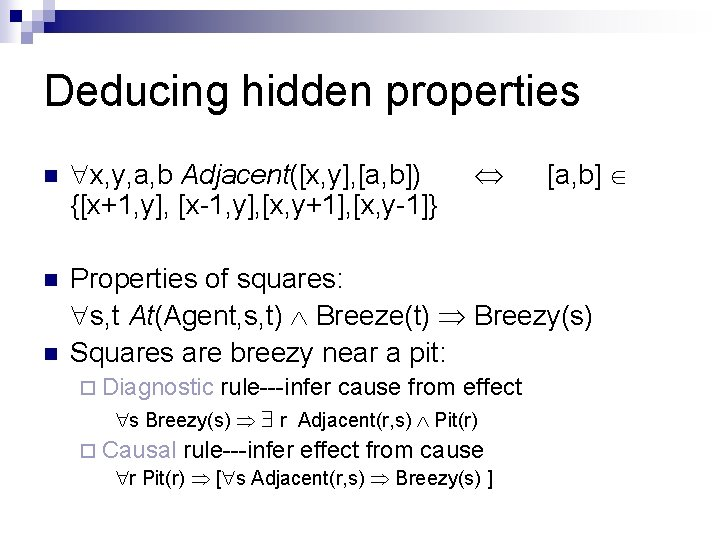Deducing hidden properties n x, y, a, b Adjacent([x, y], [a, b]) {[x+1, y], [x-1, y], [x, y+1], [x, y-1]} n Properties of squares: s, t At(Agent, s, t) Breeze(t) Breezy(s) Squares are breezy near a pit: n ¨ Diagnostic rule---infer cause from effect s Breezy(s) r Adjacent(r, s) Pit(r) ¨ Causal rule---infer effect from cause r Pit(r) [ s Adjacent(r, s) Breezy(s) ] [a, b]Knowledge engineering in FOL 1. Identify the task 1. Assemble the relevant knowledge 1. Decide on a vocabulary of predicates, functions, and constants 1. Encode general knowledge about the domain 1. Encode a description of the specific problemThe electronic circuits domain One-bit full adder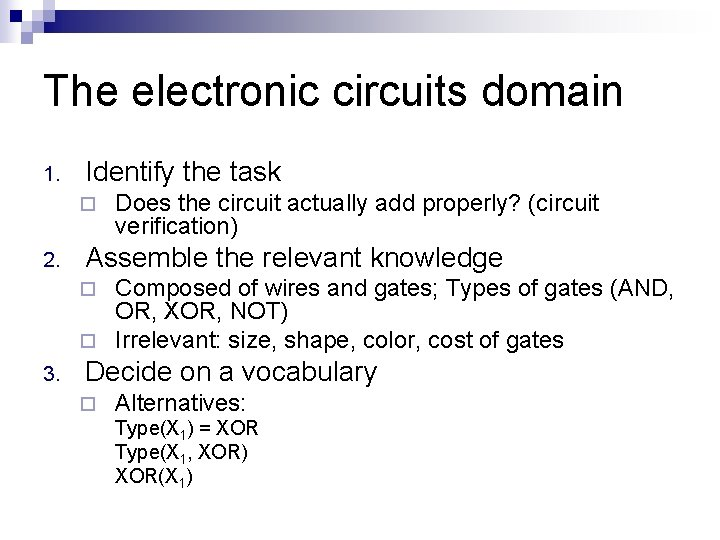The electronic circuits domain 1. Identify the task ¨ 2. Does the circuit actually add properly? (circuit verification) Assemble the relevant knowledge Composed of wires and gates; Types of gates (AND, OR, XOR, NOT) ¨ Irrelevant: size, shape, color, cost of gates ¨ 3. Decide on a vocabulary ¨ Alternatives: Type(X 1) = XOR Type(X 1, XOR) XOR(X 1)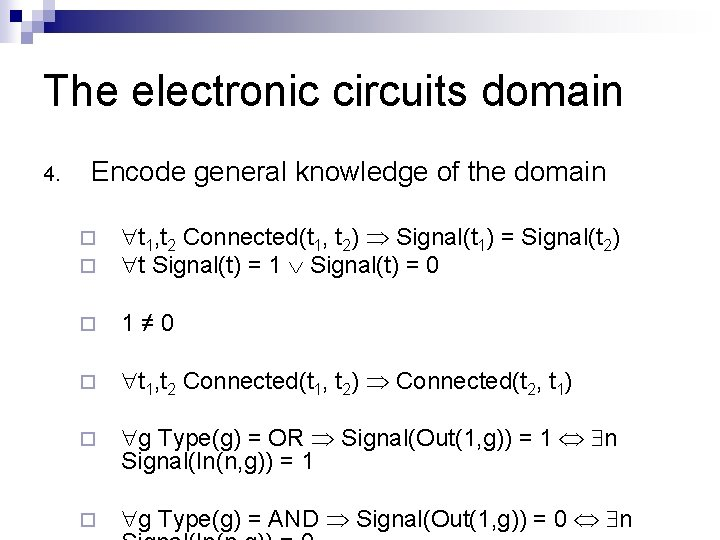The electronic circuits domain 4. Encode general knowledge of the domain ¨ ¨ t 1, t 2 Connected(t 1, t 2) Signal(t 1) = Signal(t 2) t Signal(t) = 1 Signal(t) = 0 ¨ 1≠ 0 ¨ t 1, t 2 Connected(t 1, t 2) Connected(t 2, t 1) ¨ g Type(g) = OR Signal(Out(1, g)) = 1 n Signal(In(n, g)) = 1 ¨ g Type(g) = AND Signal(Out(1, g)) = 0 n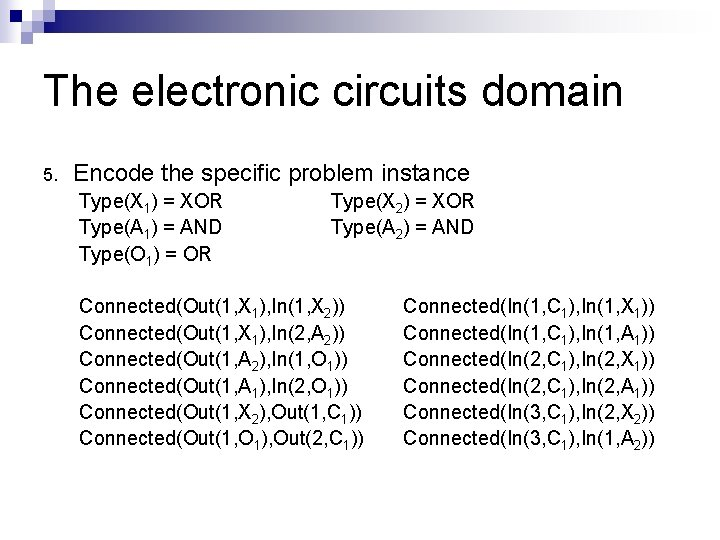The electronic circuits domain 5. Encode the specific problem instance Type(X 1) = XOR Type(A 1) = AND Type(O 1) = OR Type(X 2) = XOR Type(A 2) = AND Connected(Out(1, X 1), In(1, X 2)) Connected(Out(1, X 1), In(2, A 2)) Connected(Out(1, A 2), In(1, O 1)) Connected(Out(1, A 1), In(2, O 1)) Connected(Out(1, X 2), Out(1, C 1)) Connected(Out(1, O 1), Out(2, C 1)) Connected(In(1, C 1), In(1, X 1)) Connected(In(1, C 1), In(1, A 1)) Connected(In(2, C 1), In(2, X 1)) Connected(In(2, C 1), In(2, A 1)) Connected(In(3, C 1), In(2, X 2)) Connected(In(3, C 1), In(1, A 2))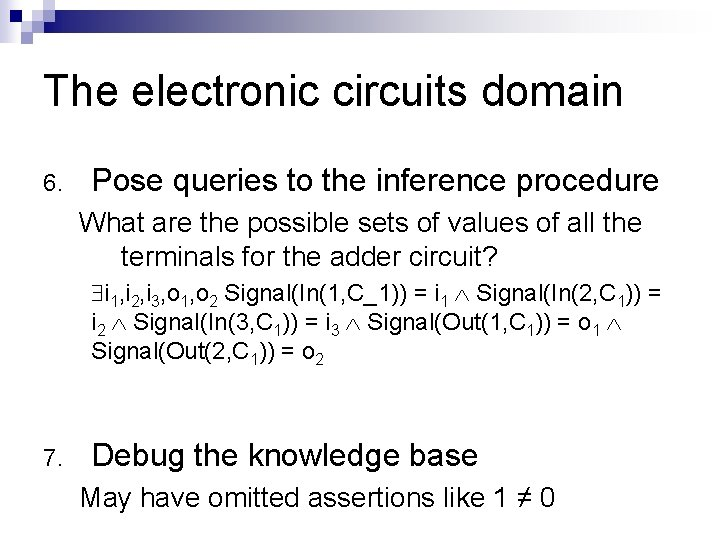The electronic circuits domain 6. Pose queries to the inference procedure What are the possible sets of values of all the terminals for the adder circuit? i 1, i 2, i 3, o 1, o 2 Signal(In(1, C_1)) = i 1 Signal(In(2, C 1)) = i 2 Signal(In(3, C 1)) = i 3 Signal(Out(1, C 1)) = o 1 Signal(Out(2, C 1)) = o 2 7. Debug the knowledge base May have omitted assertions like 1 ≠ 0Summary n First-order logic: ¨ objects and relations are semantic primitives ¨ syntax: constants, functions, predicates, equality, quantifiers n Increased expressive power: sufficient to define wumpus world